Next: Self Inductance Up: Inductance Previous: Inductance

## Mutual Inductance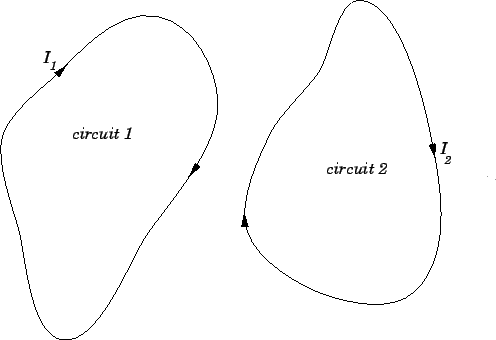Consider two arbitrary conducting circuits, labelled 1 and 2. Suppose that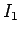is the instantaneous current flowing around circuit 1. This current generates a magnetic field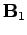which links the second circuit, giving rise to a magnetic flux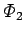through that circuit. If the currentdoubles, then the magnetic fielddoubles in strength at all points in space, so the magnetic fluxthrough the second circuit also doubles. This conclusion follows from the linearity of the laws of magnetostatics, plus the definition of magnetic flux. Furthermore, it is obvious that the flux through the second circuit is zero whenever the current flowing around the first circuit is zero. It follows that the fluxthrough the second circuit is directly proportional to the currentflowing around the first circuit. Hence, we can write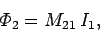(231)

where the constant of proportionality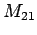is called the mutual inductance of circuit 2 with respect to circuit 1. Similarly, the flux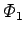through the first circuit due to the instantaneous current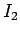flowing around the second circuit is directly proportional to that current, so we can write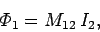(232)

where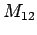is the mutual inductance of circuit 1 with respect to circuit 2. It is possible to demonstrate mathematically that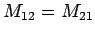. In other words, the flux linking circuit 2 when a certain current flows around circuit 1 is exactly the same as the flux linking circuit 1 when the same current flows around circuit 2. This is true irrespective of the size, number of turns, relative position, and relative orientation of the two circuits. Because of this, we can write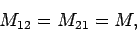(233)

where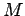is termed the mutual inductance of the two circuits. Note thatis a purely geometric quantity, depending only on the size, number of turns, relative position, and relative orientation of the two circuits. The SI units of mutual inductance are called Henries (H). One henry is equivalent to a volt-second per ampere: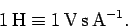(234)

It turns out that a henry is a rather unwieldy unit. The mutual inductances of the circuits typically encountered in laboratory experiments are measured in milli-henries.

Suppose that the current flowing around circuit 1 changes by an amount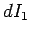in a time interval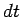. It follows from Eqs. (231) and (233) that the flux linking circuit 2 changes by an amount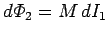in the same time interval. According to Faraday's law, an emf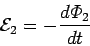(235)

is generated around the second circuit due to the changing magnetic flux linking that circuit. Since,, this emf can also be written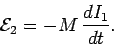(236)

Thus, the emf generated around the second circuit due to the current flowing around the first circuit is directly proportional to the rate at which that current changes. Likewise, if the currentflowing around the second circuit changes by an amount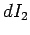in a time intervalthen the emf generated around the first circuit is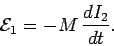(237)

Note that there is no direct physical coupling between the two circuits. The coupling is due entirely to the magnetic field generated by the currents flowing around the circuits.

As a simple example, suppose that two insulated wires are wound on the same cylindrical former, so as to form two solenoids sharing a common air-filled core. Let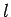be the length of the core,the cross-sectional area of the core,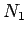the number of times the first wire is wound around the core, and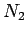the number of times the second wire is wound around the core. If a currentflows around the first wire then a uniform axial magnetic field of strength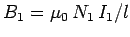is generated in the core (see Sect. 8.8). The magnetic field in the region outside the core is of negligible magnitude. The flux linking a single turn of the second wire is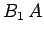. Thus, the flux linking allturns of the second wire is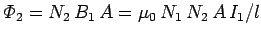. From Eq. (231), the mutual inductance of the second wire with respect to the first is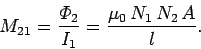(238)

Now, the flux linking the second wire when a currentflows in the first wire is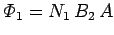, where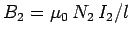is the associated magnetic field generated in the core. It follows from Eq. (232) that the mutual inductance of the first wire with respect to the second is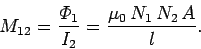(239)

Note that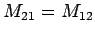, in accordance with Eq. (233). Thus, the mutual inductance of the two wires is given by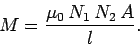(240)

As described previously,is a geometric quantity depending on the dimensions of the core, and the manner in which the two wires are wound around the core, but not on the actual currents flowing through the wires.Next: Self Inductance Up: Inductance Previous: Inductance
Richard Fitzpatrick 2007-07-14